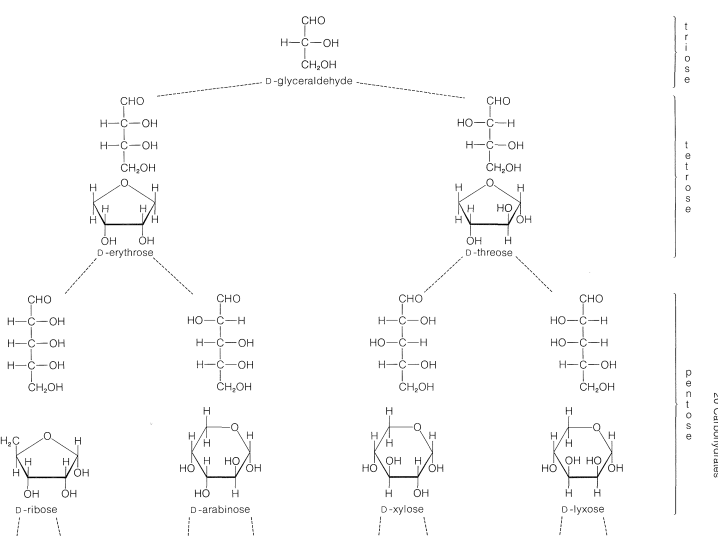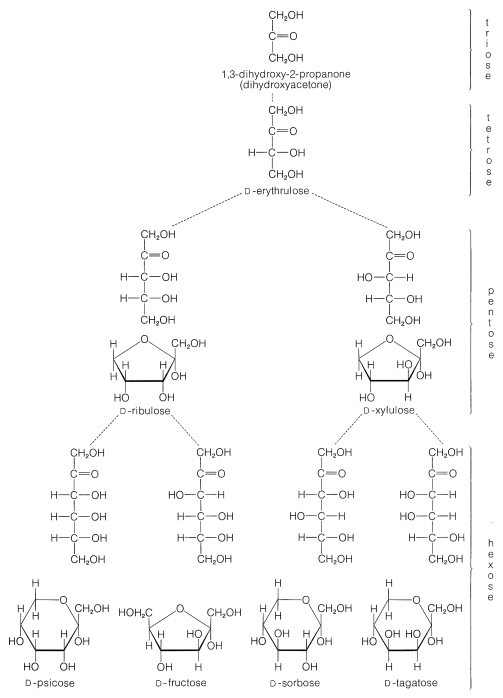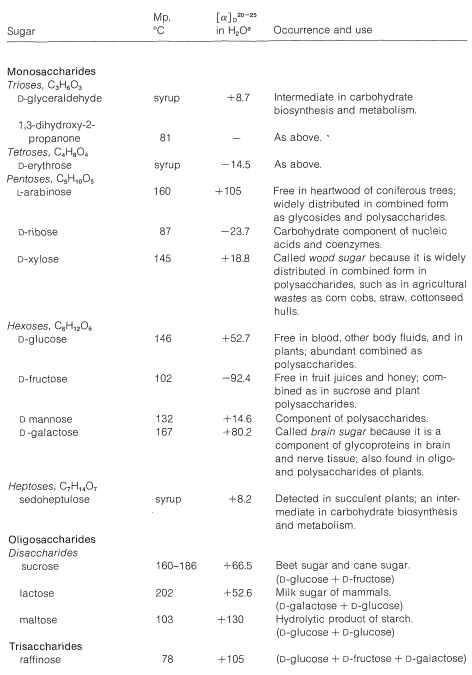# 20.2: Classification and Occurrence of Carbohydrates

$$\newcommand{\vecs}{\overset { \rightharpoonup} {\mathbf{#1}} }$$ $$\newcommand{\vecd}{\overset{-\!-\!\rightharpoonup}{\vphantom{a}\smash {#1}}}$$$$\newcommand{\id}{\mathrm{id}}$$ $$\newcommand{\Span}{\mathrm{span}}$$ $$\newcommand{\kernel}{\mathrm{null}\,}$$ $$\newcommand{\range}{\mathrm{range}\,}$$ $$\newcommand{\RealPart}{\mathrm{Re}}$$ $$\newcommand{\ImaginaryPart}{\mathrm{Im}}$$ $$\newcommand{\Argument}{\mathrm{Arg}}$$ $$\newcommand{\norm}{\| #1 \|}$$ $$\newcommand{\inner}{\langle #1, #2 \rangle}$$ $$\newcommand{\Span}{\mathrm{span}}$$ $$\newcommand{\id}{\mathrm{id}}$$ $$\newcommand{\Span}{\mathrm{span}}$$ $$\newcommand{\kernel}{\mathrm{null}\,}$$ $$\newcommand{\range}{\mathrm{range}\,}$$ $$\newcommand{\RealPart}{\mathrm{Re}}$$ $$\newcommand{\ImaginaryPart}{\mathrm{Im}}$$ $$\newcommand{\Argument}{\mathrm{Arg}}$$ $$\newcommand{\norm}{\| #1 \|}$$ $$\newcommand{\inner}{\langle #1, #2 \rangle}$$ $$\newcommand{\Span}{\mathrm{span}}$$$$\newcommand{\AA}{\unicode[.8,0]{x212B}}$$

The simple sugars, or monosaccharides, are the building blocks of carbohydrate chemistry. They are polyhydroxy aldehydes or ketones with five, six, seven, or eight carbon atoms that are classified appropriately as pentoses, hexoses, heptoses, or octoses, respectively. They can be designated by more specific names, such as aldohexose or ketohexose, to denote the kind of carbonyl compound they represent. For example, and aldopentose is a five-carbon sugar with an aldehyde carbonyl; a ketohexose is a six-carbon sugar with a ketone carbonyl:However, it is important to keep in mind that the carbonyl groups of sugars usually are combined with one of the hydroxyl groups in the same molecule to form a cyclic hemiacetal or hemiketal. These structures once were written as follows, and considerable stretch of the imagination is needed to recognize that they represent oxacycloalkane ring systems:The saccharides have long and awkward names by the IUPAC system, consequently a highly specialized nomenclature system has been developed for carbohydrates. Because this system (and those like it for other natural products) is unlikely to be replaced by more systematic names, you will find it necessary to memorize some names and structures. It will help you to remember the meaning of names such as aldopentose and ketohexose, and to learn the names and details of the structures of glucose, fructose, and ribose. For the rest of the carbohydrates, the nonspecialist needs only to remember the kind of compounds that they are.

The most abundant five-carbon sugars are $$L$$-arabinose, $$D$$-ribose, 2-deoxy-$$D$$-ribose,$$^1$$ and $$D$$-xylose, which all are aldopentoses. Both the open-chain and cyclic structures of the $$D$$-aldoses up to $$\ce{C_6}$$ are shown in Figure 20-1.Figure 20-1: Structure and configuration of the $$D$$-aldoses from $$\ce{C_3}$$ to $$\ce{C_6}$$, showing the configurational relationship to $$D$$-glyceraldehyde. Open-chain and cyclic forms are shown. The oxacyclohexane (pyranose) form is more stable than the oxacyclopentane (furanose) form for the free sugar. The oxacyclopentane structure is shown for ribose because this is the form in which is occurs in many important substance, such as the nucleic acids. Only the $$\alpha$$ anomers are shown (see Section 20-2B).

The common six-carbon sugars (hexoses) are $$D$$-glucose, $$D$$-fructose, $$D$$-galactose, and $$D$$-mannose. They all are aldohexoses, except $$D$$-fructose, which is a ketohexose. The structures of the ketoses up to $$\ce{C_6}$$ are shown for reference in Figure 20-2. The occurrence and uses of the more important ketoses and aldoses are summarized in Table 20-1.Figure 20-1), the cyclic form is predominantly an oxacyclohexane (pyranose) ring in the free sugar, but the oxacyclopentane (furanose) form is shown because it occurs widely in this form as the disaccharide, sucrose. Only the $$\alpha$$ anomers are shown (see Section 20-2B).

Table 20-1: Occurrence, Physical Properties, and Uses of Some Natural Sugars$$^1$$Deoxy means lacking a hydroxyl group, and 2-deoxyribose is simply ribose without an $$\ce{OH}$$ group at the 2-carbon.